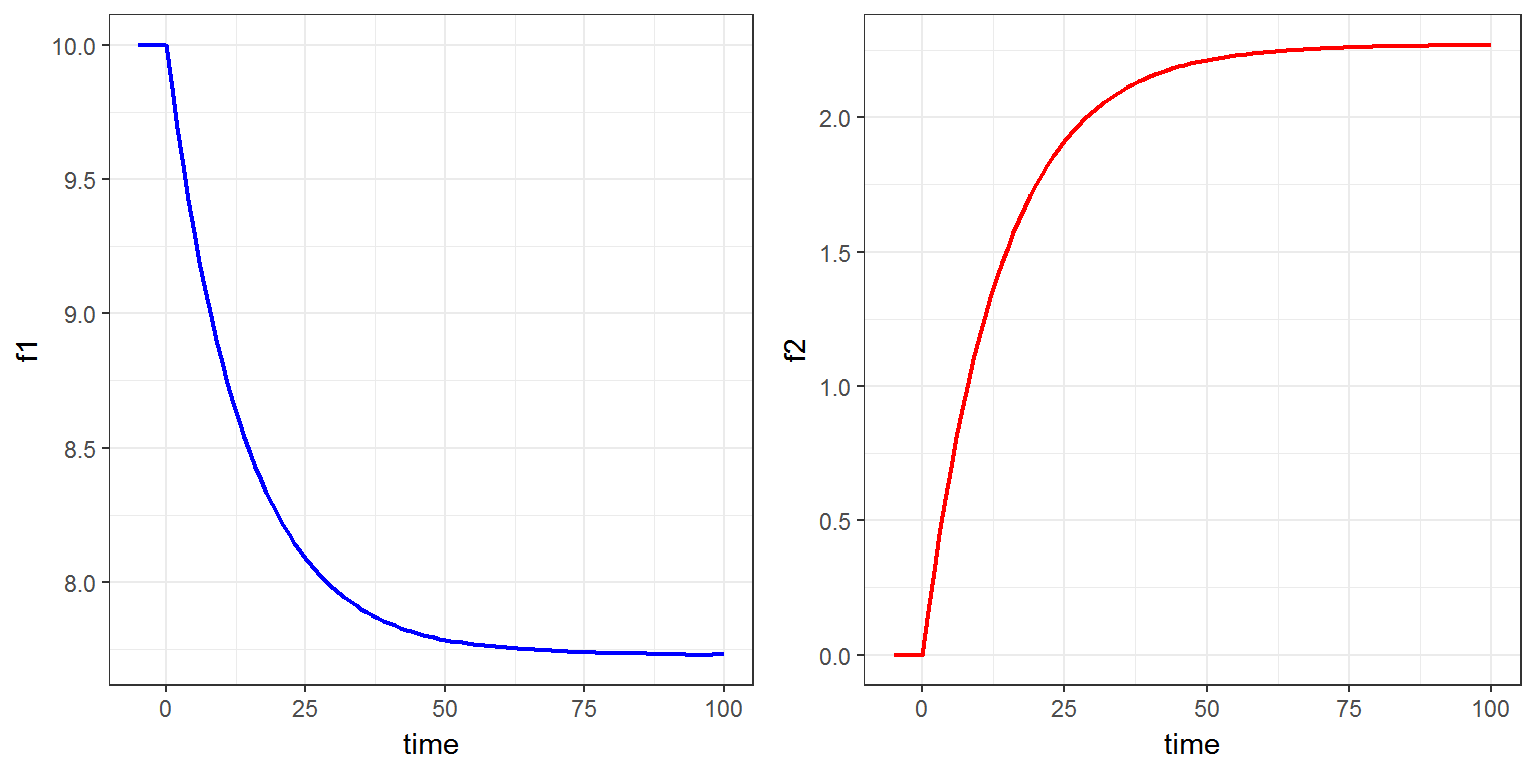R script: ode.R

$$\newcommand{\esp}{\mathbb{E}\left(#1\right)} \newcommand{\var}{\mbox{Var}\left(#1\right)} \newcommand{\deriv}{\dot{#1}(t)} \newcommand{\prob}{ \mathbb{P}\!(#1)} \newcommand{\eqdef}{\mathop{=}\limits^{\mathrm{def}}} \newcommand{\by}{\boldsymbol{y}} \newcommand{\bc}{\boldsymbol{c}} \newcommand{\bpsi}{\boldsymbol{\psi}} \def\pmacro{\texttt{p}} \def\like{{\cal L}} \def\llike{{\cal LL}} \def\logit{{\rm logit}} \def\probit{{\rm probit}} \def\one{{\rm 1\!I}} \def\iid{\mathop{\sim}_{\rm i.i.d.}} \def\simh0{\mathop{\sim}_{H_0}} \def\df{\texttt{df}} \def\res{e} \def\xomega{x} \newcommand{\argmin}{{\rm arg}\min_{#1}} \newcommand{\argmax}{{\rm arg}\max_{#1}} \newcommand{\Rset}{\mbox{\mathbb{R}}} \def\param{\theta} \def\setparam{\Theta} \def\xnew{x_{\rm new}} \def\fnew{f_{\rm new}} \def\ynew{y_{\rm new}} \def\nnew{n_{\rm new}} \def\enew{e_{\rm new}} \def\Xnew{X_{\rm new}} \def\hfnew{\widehat{\fnew}} \def\degree{m} \def\nbeta{d} \newcommand{\limite}{\mathop{\longrightarrow}\limits_{#1}} \def\ka{k{a}} \def\ska{k{\scriptscriptstyle a}} \def\kel{k{e}} \def\skel{k{\scriptscriptstyle e}} \def\cl{C{\small l}} \def\Tlag{T\hspace{-0.1em}{lag}} \def\sTlag{T\hspace{-0.07em}{\scriptscriptstyle lag}} \def\Tk{T\hspace{-0.1em}{k0}} \def\sTk{T\hspace{-0.07em}{\scriptscriptstyle k0}} \def\thalf{t{1/2}} \newcommand{\Dphi}{\partial_\pphi #1} \def\asigma{a} \def\pphi{\psi} \newcommand{\stheta}{{\theta^\star}} \newcommand{\htheta}{{\widehat{\theta}}}$$

# 1 Introduction

A system of ordinary differential equations (ODEs) can be implemented in a block EQUATION of the section [LONGITUDINAL] of a script Mlxtran.

The differentiation variable is $$t$$.

The problem is defined by the equations of the derivatives, the initial time point and the initial values of the components. The stiffness of the problem can be defined. The problem accepts input source terms, which allows to bring some discontinuity into the defined derivatives.

## 1.1 Derivatives

The keywords prefixed with ddt_ in front of a variable name, as ddt_a and ddt_b, define the derivatives of an ODE system. The variable names denote the components of the solution. These variables are defined at the whole section level through their derivatives. The derivatives themselves aren’t variables but keywords, and cannot be referenced by other equations nor be defined under a conditional statement.

The structure of the ODE system is part of the structure of the whole model and cannot be conditional. Adding conditional intermediate variables for the values of the derivatives easily provides this flexibility.

## 1.2 Initial values

The keyword t0 defines the initial point $$t_0$$ of an ODE problem, while the variable names with suffix _0 define the initial values of the system. More precisely, the components are defined for $$t \leq t_0$$ as the equations provided for the initial values, and for $$t > t_0$$ they are defined as the solution of the ODE system with initial values fixed at $$t = t_0$$.

Initial values cannot depend on $$t$$, but can be functions of $$t_0$$. By default, an initial value is null.

For example, the following model \begin{align} \deriv{x} &= \frac{{\rm d}\, x}{{\rm d}\, t}(t) = -k\, x^c, \quad \text{for } \ \ t \geq 5\\ x(t) &= x_0, \quad \text{for } \ \ t \leq 5 \end{align} is implemented as follows with Mlxtran:
t0 = 5
x_0 = x0
ddt_x = - k*x^c


## 1.3 Type of ODE’s

The keyword odeType defines the type of the ODE system. The problem can be indicated as being stiff, non-stiff, or linear. By default, the problem is viewed as non-stiff.

odeType = stiff
; Other possible values:
; odeType = nonStiff
; odeType = linear


# 2 Example

We consider in this example a model where two functions $$f_1$$ and $$f_2$$ depending on a vector of parameters $$\phi=(a, b, c)$$ are defined by a system of ODEs

\begin{align} \deriv{f_1} &= a\, f_2(t) - \frac{b\, f_1(t)}{1 + c\,f_1(t)} \\ \deriv{f_2} &= \frac{b\, f_1(t)}{1 + c\,f_1(t)} - a\, f_2(t) \end{align}

with the following initial condition: \begin{align} f_1(t) &= 10 \quad {\rm for } \ t\leq 0 \\ f_2(t) &= 0 \ \ \quad {\rm for } \ t\leq 0 \end{align}

Here, this model is implemented as an inline model in the R script (we could use equivalently the model file model/ode.txt):

ode.model <- inlineModel("
[LONGITUDINAL]
input = {a, b, c}

EQUATION:
t0     = 0
f1_0   = 10
f2_0   = 0
ddt_f1 = a*f2 - b*f1/(1+c*f1)
ddt_f2 = b*f1/(1+c*f1) - a*f2
")

We will use simulx for evaluating $$f_1$$ and $$f_2$$ every hour between time -5h and time 100h, with $$a=0.07$$, $$b=0.1$$ and $$c=0.5$$:

p <- c(a = 0.07, b = 0.1, c = 0.5)

out <- list(name=c('f1', 'f2'), time=-5:100)

res <- simulx(model     = ode.model,
parameter = p,
output    = out)

res is a list with two fields f1 and f2 which are two data frames with 106 rows and 2 columns.

We can now plot this data:

library(gridExtra)
plot1=ggplot(data=res$f1, aes(x=time, y=f1)) + geom_line(colour='blue',size=0.75) plot2=ggplot(data=res$f2, aes(x=time, y=f2)) + geom_line(colour='red',size=0.75)
grid.arrange(plot1, plot2, ncol=2)`